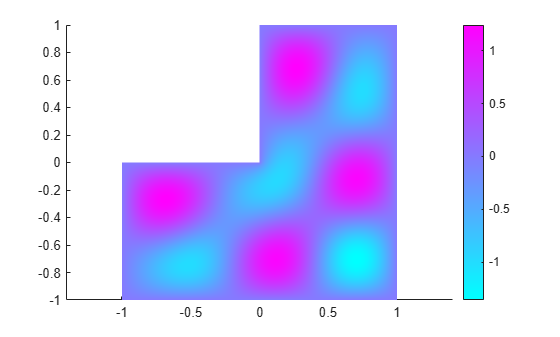Main Content

# EigenResults

PDE eigenvalue solution and derived quantities

## Description

An `EigenResults` object contains the solution of a PDE eigenvalue problem in a form convenient for plotting and postprocessing.

• Eigenvector values at the nodes appear in the `Eigenvectors` property.

• The eigenvalues appear in the `Eigenvalues` property.

## Creation

There are several ways to create an `EigenResults` object:

• Solve an eigenvalue problem using the `solvepdeeig` function. This function returns a PDE eigenvalue solution as an `EigenResults` object. This is the recommended approach.

• Solve an eigenvalue problem using the `pdeeig` function. Then use the `createPDEResults` function to obtain an `EigenResults` object from a PDE eigenvalue solution returned by `pdeeig`. Note that `pdeeig` is a legacy function. It is not recommended for solving eigenvalue problems.

## Properties

expand all

Finite element mesh, returned as a FEMesh Properties object.

Solution eigenvectors, returned as a matrix or 3-D array. The solution is a matrix for scalar eigenvalue problems, and a 3-D array for eigenvalue systems. For details, see Dimensions of Solutions, Gradients, and Fluxes.

Data Types: `double`

Solution eigenvalues, returned as a vector. The vector is in order by the real part of the eigenvalues from smallest to largest.

Data Types: `double`

## Object Functions

 `interpolateSolution` Interpolate PDE solution to arbitrary points

## Examples

collapse all

Obtain an `EigenResults` object from `solvepdeeig`.

Create the geometry for the L-shaped membrane. Apply zero Dirichlet boundary conditions to all edges.

```model = createpde; geometryFromEdges(model,@lshapeg); applyBoundaryCondition(model,'dirichlet','Edge',1:model.Geometry.NumEdges,'u',0);```

Specify coefficients `c = 1`, `a = 0`, and `d = 1`.

`specifyCoefficients(model,'m',0,'d',1,'c',1,'a',0,'f',0);`

Create the mesh and solve the eigenvalue problem for eigenvalues from 0 through 100.

```generateMesh(model,'Hmax',0.05); ev = [0,100]; results = solvepdeeig(model,ev)```
``` Basis= 10, Time= 0.17, New conv eig= 0 Basis= 11, Time= 0.19, New conv eig= 0 Basis= 12, Time= 0.19, New conv eig= 0 Basis= 13, Time= 0.20, New conv eig= 0 Basis= 14, Time= 0.21, New conv eig= 0 Basis= 15, Time= 0.22, New conv eig= 0 Basis= 16, Time= 0.22, New conv eig= 0 Basis= 17, Time= 0.23, New conv eig= 0 Basis= 18, Time= 0.23, New conv eig= 1 Basis= 19, Time= 0.23, New conv eig= 1 Basis= 20, Time= 0.23, New conv eig= 1 Basis= 21, Time= 0.24, New conv eig= 1 Basis= 22, Time= 0.24, New conv eig= 3 Basis= 23, Time= 0.24, New conv eig= 3 Basis= 24, Time= 0.25, New conv eig= 4 Basis= 25, Time= 0.26, New conv eig= 5 Basis= 26, Time= 0.26, New conv eig= 6 Basis= 27, Time= 0.27, New conv eig= 6 Basis= 28, Time= 0.27, New conv eig= 6 Basis= 29, Time= 0.27, New conv eig= 7 Basis= 30, Time= 0.28, New conv eig= 7 Basis= 31, Time= 0.28, New conv eig= 10 Basis= 32, Time= 0.29, New conv eig= 10 Basis= 33, Time= 0.29, New conv eig= 11 Basis= 34, Time= 0.29, New conv eig= 11 Basis= 35, Time= 0.30, New conv eig= 14 Basis= 36, Time= 0.30, New conv eig= 14 Basis= 37, Time= 0.30, New conv eig= 14 Basis= 38, Time= 0.31, New conv eig= 14 Basis= 39, Time= 0.31, New conv eig= 14 Basis= 40, Time= 0.32, New conv eig= 14 Basis= 41, Time= 0.33, New conv eig= 15 Basis= 42, Time= 0.33, New conv eig= 15 Basis= 43, Time= 0.33, New conv eig= 15 Basis= 44, Time= 0.35, New conv eig= 16 Basis= 45, Time= 0.62, New conv eig= 16 Basis= 46, Time= 0.64, New conv eig= 16 Basis= 47, Time= 0.64, New conv eig= 16 Basis= 48, Time= 0.66, New conv eig= 17 Basis= 49, Time= 0.67, New conv eig= 18 Basis= 50, Time= 0.69, New conv eig= 18 Basis= 51, Time= 0.71, New conv eig= 18 Basis= 52, Time= 0.73, New conv eig= 18 Basis= 53, Time= 0.73, New conv eig= 18 Basis= 54, Time= 0.74, New conv eig= 21 End of sweep: Basis= 54, Time= 0.74, New conv eig= 21 Basis= 31, Time= 0.79, New conv eig= 0 Basis= 32, Time= 0.83, New conv eig= 0 Basis= 33, Time= 0.84, New conv eig= 0 End of sweep: Basis= 33, Time= 0.85, New conv eig= 0 ```
```results = EigenResults with properties: Eigenvectors: [5597x19 double] Eigenvalues: [19x1 double] Mesh: [1x1 FEMesh] ```

Plot the solution for mode 10.

`pdeplot(model,'XYData',results.Eigenvectors(:,10))`## See Also

Introduced in R2016a

## SupportGet trial now